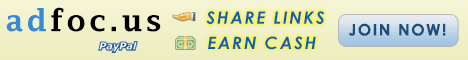WELCOME TO MY SITE, In All Your Troubles And Agonies Be Patient And Trust In The Goodness of ALLAH Who Alone Can Relieve You And Give Real Happiness And Peace. AL-QURAN. http://www.technodesh.com, http://www.engineerjamal.com, http://picturemuseum.blogspot.com and More...

# How to Convert Office Excel Numeric Data into English Words

How to create the sample function Called SpellNumber:
1. Start Microsoft Excel
1. Press ALT+F11 to start the Visual Basic Editor.
2. On the Insert menu, click Module.
3. Type the following code into the module sheet.
Option Explicit
'Main Function
Function SpellNumber(ByVal MyNumber)
Dim Dollars, Cents, Temp
Dim DecimalPlace, Count
ReDim Place(9) As String
Place(2) = " Thousand "
Place(3) = " Million "
Place(4) = " Billion "
Place(5) = " Trillion "
' String representation of amount.
MyNumber = Trim(Str(MyNumber))
' Position of decimal place 0 if none.
DecimalPlace = InStr(MyNumber, ".")
' Convert cents and set MyNumber to dollar amount.
If DecimalPlace > 0 Then
Cents = GetTens(Left(Mid(MyNumber, DecimalPlace + 1) & _
"00", 2))
MyNumber = Trim(Left(MyNumber, DecimalPlace - 1))
End If
Count = 1
Do While MyNumber <> ""
Temp = GetHundreds(Right(MyNumber, 3))
If Temp <> "" Then Dollars = Temp & Place(Count) & Dollars
If Len(MyNumber) > 3 Then
MyNumber = Left(MyNumber, Len(MyNumber) - 3)
Else
MyNumber = ""
End If
Count = Count + 1
Loop
Select Case Dollars
Case ""
Dollars = "No Dollars"
Case "One"
Dollars = "One Dollar"
Case Else
Dollars = Dollars & " Dollars"
End Select
Select Case Cents
Case ""
Cents = " and No Cents"
Case "One"
Cents = " and One Cent"
Case Else
Cents = " and " & Cents & " Cents"
End Select
SpellNumber = Dollars & Cents
End Function

' Converts a number from 100-999 into text
Function GetHundreds(ByVal MyNumber)
Dim Result As String
If Val(MyNumber) = 0 Then Exit Function
MyNumber = Right("000" & MyNumber, 3)
' Convert the hundreds place.
If Mid(MyNumber, 1, 1) <> "0" Then
Result = GetDigit(Mid(MyNumber, 1, 1)) & " Hundred "
End If
' Convert the tens and ones place.
If Mid(MyNumber, 2, 1) <> "0" Then
Result = Result & GetTens(Mid(MyNumber, 2))
Else
Result = Result & GetDigit(Mid(MyNumber, 3))
End If
GetHundreds = Result
End Function

' Converts a number from 10 to 99 into text.
Function GetTens(TensText)
Dim Result As String
Result = ""           ' Null out the temporary function value.
If Val(Left(TensText, 1)) = 1 Then   ' If value between 10-19...
Select Case Val(TensText)
Case 10: Result = "Ten"
Case 11: Result = "Eleven"
Case 12: Result = "Twelve"
Case 13: Result = "Thirteen"
Case 14: Result = "Fourteen"
Case 15: Result = "Fifteen"
Case 16: Result = "Sixteen"
Case 17: Result = "Seventeen"
Case 18: Result = "Eighteen"
Case 19: Result = "Nineteen"
Case Else
End Select
Else                                 ' If value between 20-99...
Select Case Val(Left(TensText, 1))
Case 2: Result = "Twenty "
Case 3: Result = "Thirty "
Case 4: Result = "Forty "
Case 5: Result = "Fifty "
Case 6: Result = "Sixty "
Case 7: Result = "Seventy "
Case 8: Result = "Eighty "
Case 9: Result = "Ninety "
Case Else
End Select
Result = Result & GetDigit _
(Right(TensText, 1))  ' Retrieve ones place.
End If
GetTens = Result
End Function

' Converts a number from 1 to 9 into text.
Function GetDigit(Digit)
Select Case Val(Digit)
Case 1: GetDigit = "One"
Case 2: GetDigit = "Two"
Case 3: GetDigit = "Three"
Case 4: GetDigit = "Four"
Case 5: GetDigit = "Five"
Case 6: GetDigit = "Six"
Case 7: GetDigit = "Seven"
Case 8: GetDigit = "Eight"
Case 9: GetDigit = "Nine"
Case Else: GetDigit = ""
End Select
End Function
How to use the SpellNumber sample function:
To use the sample functions to change a number to written text, use one of the methods demonstrated in the following examples:

Method 1: Direct Entry
You can change 32.50 into "Thirty Two Dollars and Fifty Cents" by entering the following formula into a cell:
=SpellNumber(32.50)

Method 2: Cell reference
You can refer to other cells in the workbook. For example, enter the number 32.50 into cell A1, and type the following formula into another cell:
=SpellNumber(A1)

Method 3: Paste Function or Insert Function
To enter a custom function into a worksheet, you can use Paste Function in Excel 2000, or you can use Insert Function in Excel 2002 and in Excel 2003.
Excel 2000:
1. To use Paste Function, follow these steps:
2. Select the cell that you want.
3. Click Paste Function on the Standard toolbar.
4. Under Function category, click User Defined.
5. Under Function name, click SpellNumber, and then click OK.
6. Enter the number or cell reference that you want, and then click OK.
Excel 2002 and Excel 2003:
1. To use Insert Function, follow these steps:
2. Select the cell that you want.
3. Click Insert Function on the Standard toolbar.
4. Under Or select a category, click User Defined.
5. In the Select a function list, click SpellNumber, and then click OK.
6. Enter the number or cell reference that you want, and then click OK.
Properties:
Article ID: 213360 - Last Review: June 19, 2007 - Revision: 7.4

Applies To:
Microsoft Office Excel 2003
Microsoft Excel 2002 Standard Edition
Microsoft Excel 2000 Standard Edition
Microsoft Excel 97 Standard Edition

Summary:
This article contains sample Microsoft Visual Basic for Applications functions that you can use to convert a numeric value in a Microsoft Excel worksheet cell into its equivalent in English words.